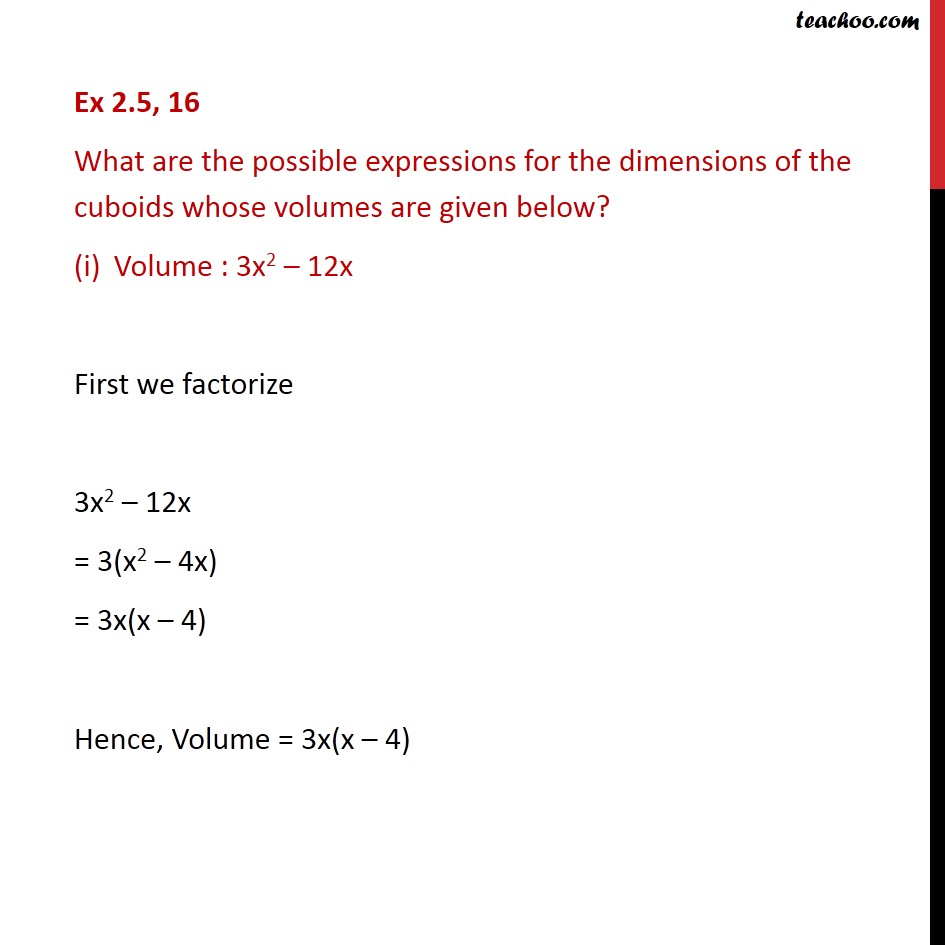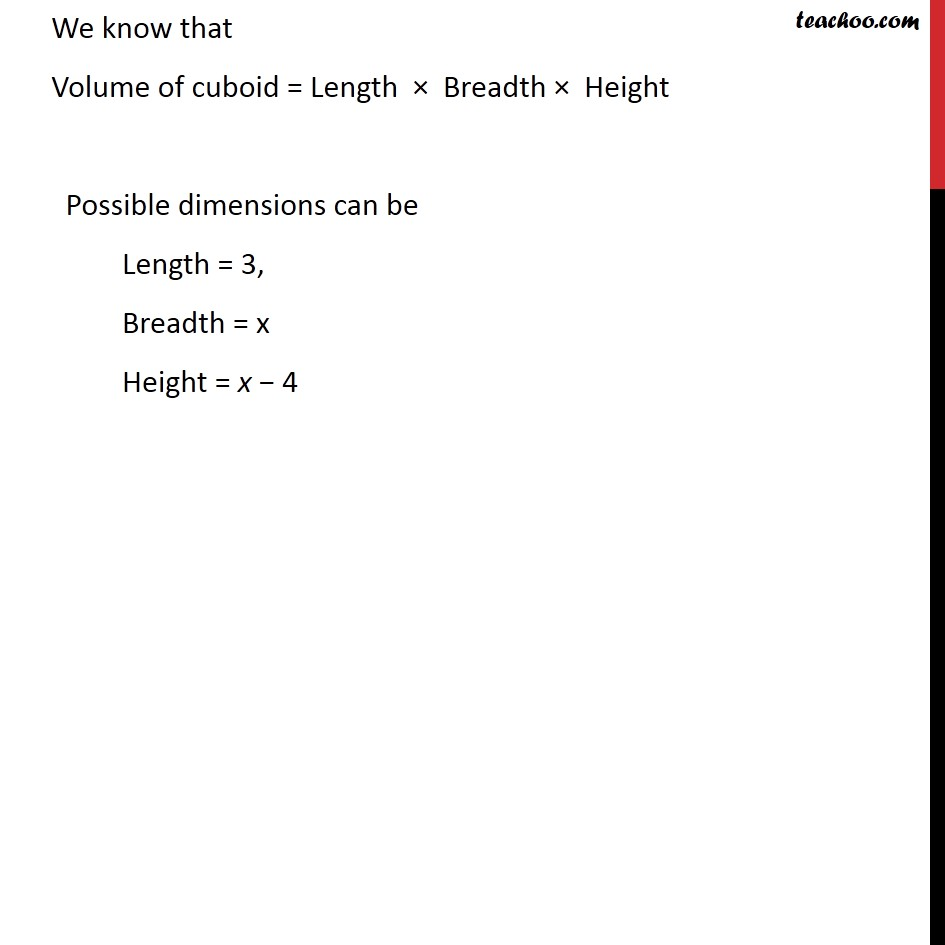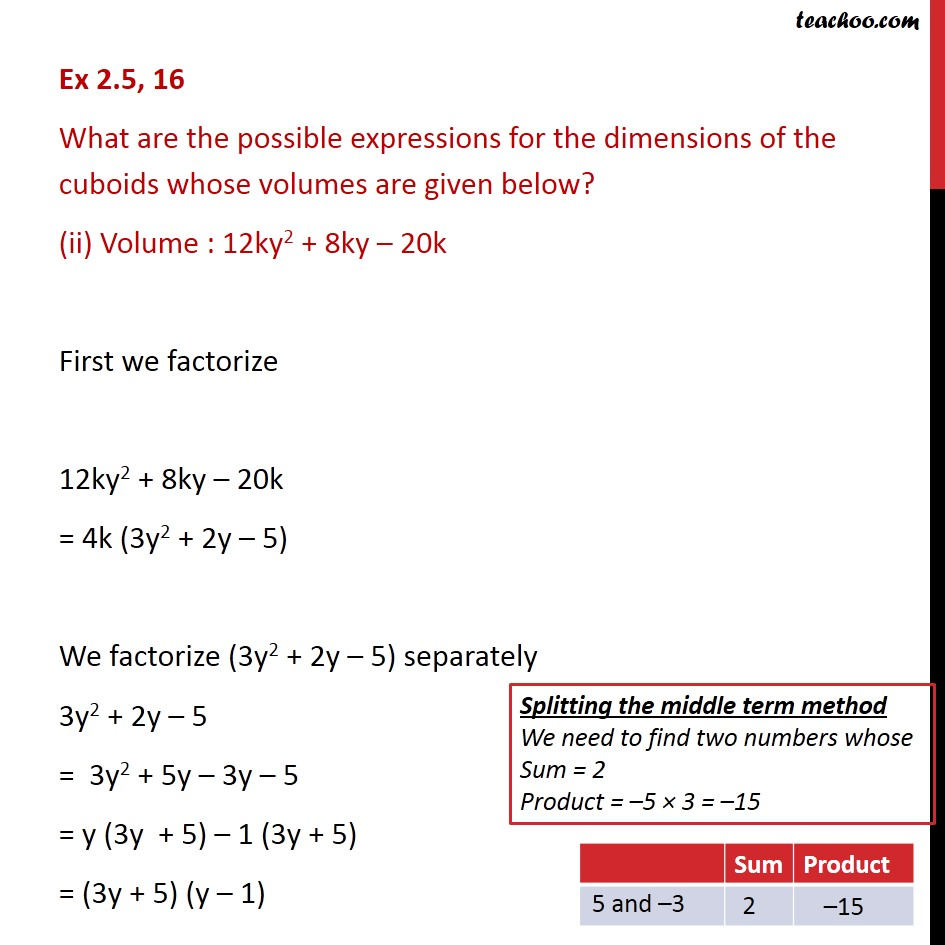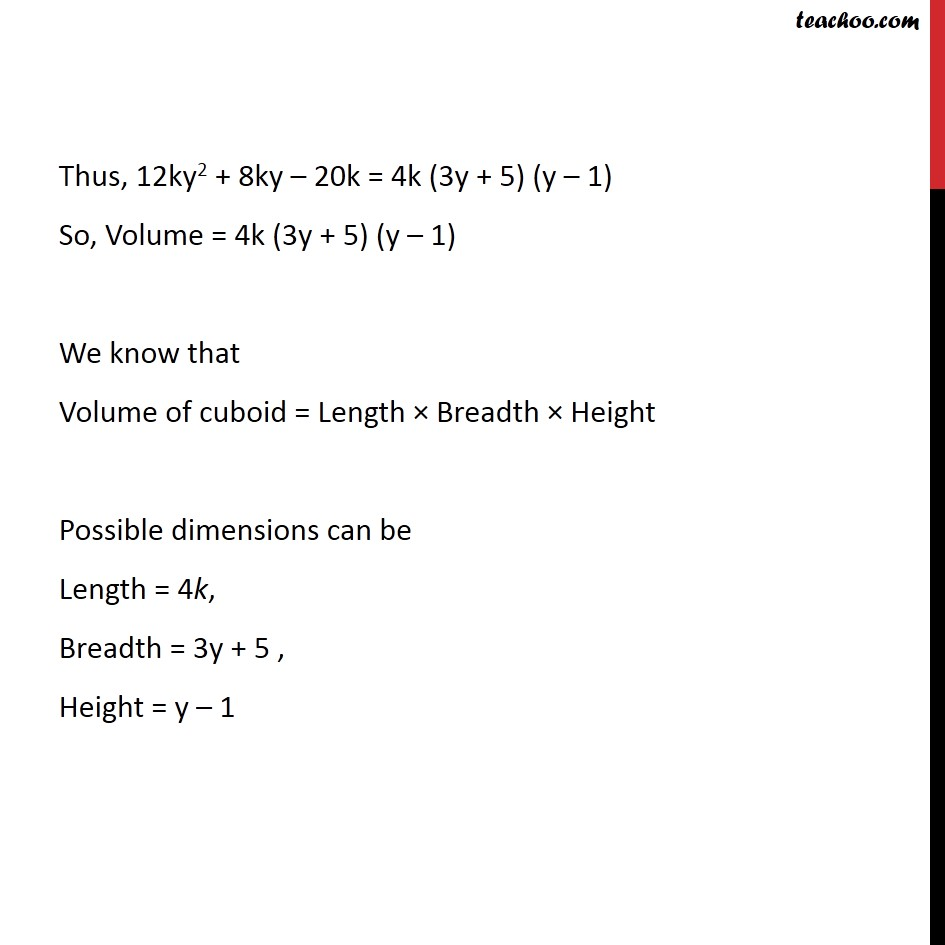Learn all Concepts of Polynomials Class 9 (with VIDEOS). Check - Polynomials Class 91. Chapter 2 Class 9 Polynomials
2. Serial order wise
3. Ex 2.5

Transcript

Ex 2.5, 16 What are the possible expressions for the dimensions of the cuboids whose volumes are given below? Volume : 3x2 – 12x First we factorize 3x2 – 12x = 3(x2 – 4x) = 3x(x – 4) Hence, Volume = 3x(x – 4) We know that Volume of cuboid = Length × Breadth × Height Possible dimensions can be Length = 3, Breadth = x Height = x − 4 Ex 2.5, 16 What are the possible expressions for the dimensions of the cuboids whose volumes are given below? (ii) Volume : 12ky2 + 8ky – 20k First we factorize 12ky2 + 8ky – 20k = 4k (3y2 + 2y – 5) We factorize (3y2 + 2y – 5) separately 3y2 + 2y – 5 = 3y2 + 5y – 3y – 5 = y (3y + 5) – 1 (3y + 5) = (3y + 5) (y – 1) Thus, 12ky2 + 8ky – 20k = 4k (3y + 5) (y – 1) So, Volume = 4k (3y + 5) (y – 1) We know that Volume of cuboid = Length × Breadth × Height Possible dimensions can be Length = 4k, Breadth = 3y + 5 , Height = y – 1

Ex 2.5

Chapter 2 Class 9 Polynomials
Serial order wise